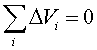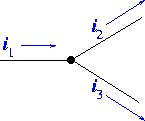Kirchoff's laws

There are two laws necessary for solving circuit problems. For simple circuits,we have been applying these equations almost instinctively.

1. The voltages around a closed path in a circuit must sum to zero. (Kirchoff's law #1), the voltage drops being negative (following a current through a resistor), while the gains are positive (going through a battery from the negative to the positive terminal).

2. The sum of the currents entering a node must equal the sum of the currents exiting a node. (Kirchoff's Law #2)

The first law is a simple statement of the meaning of potential. Since every point on a circuit has a unique value of the potential, travelling around the circuit, through any path must bring you back to the potential. Using the analogy to elevation: If one hikes from a starting point of a mountain, taking several paths, then finishes at the same point, the sum of the elevation changes of each path had better add to zero.The second law is the statment of current conservation mentioned before in the Ohm's law lecture. For the node on the right, i1=i2+i3. If all currents had been defined as enterning the node, then the sum of the currents would be zero.